Next: Explanation of the Hard Up: The Trust-Region subproblem Previous: The Trust-Region subproblem   Contents

#must be positive definite.

The solution we are seeking lies either interior to the trust region () or on the boundary.
If it lies on the interior, the trust region may as well not have been there and therefore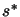is the unconstrained minimizer of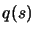. We have seen in Equation 2.4 (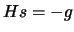) how to find it. We have seen in Equation 2.6 that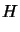must be definite positive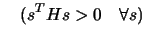(4.2)

in order to be able to apply 2.4.
If we found a value ofusing 2.4 which lies outside the trust region, it means thatlies on the trust region boundary. Let's take a closer look to this case:
Theorem 1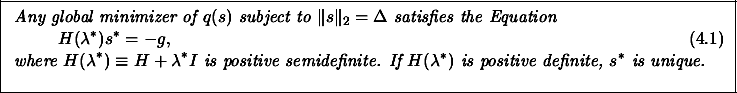First, we rewrite the constraints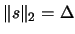as. Now, we introduce a Lagrange multiplier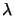for the constraint and use first-order optimality conditions (see Annexe, section 13.3 ). This gives: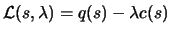(4.3)

Using first part of Equation 13.22,we have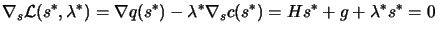(4.4)

which is 4.3.
We will now proof thatmust be positive (semi)definite.
Suppose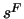is a feasible point (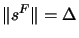), we obtain:(4.5)

Using the secant equation (see Annexes, Section 13.4),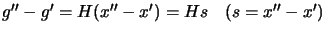, we can rewrite 4.5 into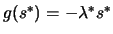. This and the restriction thatimplies that: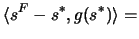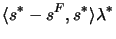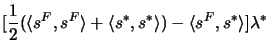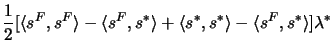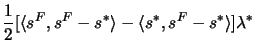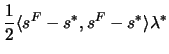(4.6)

Combining 4.6 and 4.7(4.7)

Let's define a line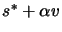as a function of the scalar. This line intersect the constraintsfor two values of: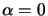and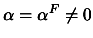at which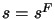. So, and therefore, using 4.8, we have that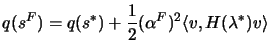Finally, as we are assuming thatis a global minimizer, we must have that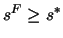, and thus that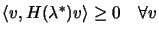, which is the same as saying that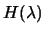is positive semidefinite.
Ifis positive definite, then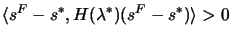for any, and therefore 4.8 shows thatwheneveris feasible. Thusis the unique global minimizer.
Using 4.2 (which is concerned about an interior minimizer) and the previous paragraph (which is concerned about a minimizer on the boundary of the trust region), we can state:
Theorem 2: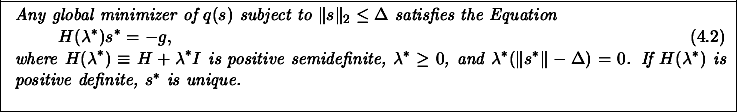The justification ofis simply the complementarity condition (see Section 13.3 for explanation, Equation 13.22).
The parameteris said to regularized'' or modify'' the model such that the modified model is convex and so that its minimizer lies on or within the trust region boundary.Next: Explanation of the Hard Up: The Trust-Region subproblem Previous: The Trust-Region subproblem   Contents
Frank Vanden Berghen 2004-04-19